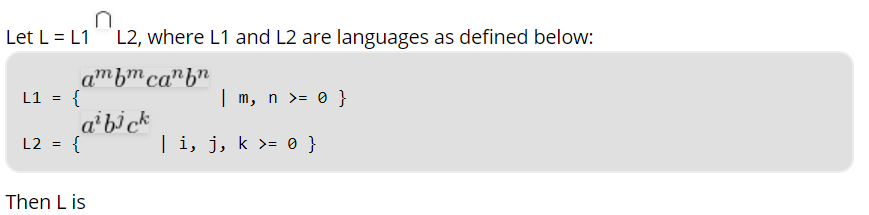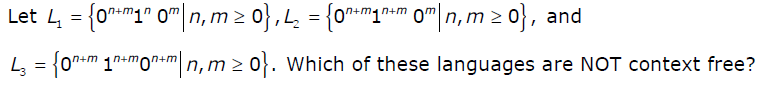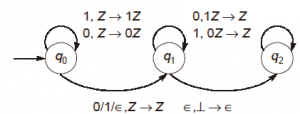A directory of Objective Type Questions covering all the Computer Science subjects. Here you can access and discuss Multiple choice questions and answers for various compitative exams and interviews.

#### Important Notice!

Dear users, compscibits.com is now permanently moved to compsciedu.com. Please update all your links and bookmarks accordingly. Soon, the site will be accessible through URL compsciedu.com only.

 91. S -> aSa|bSb|a|b; The language generated by the above grammar over the alphabet {a,b} is the set of a. All palindromes b. All odd length palindromes c. Strings that begin and end with the same symbol d. All even length palindromes

 92. Consider the below question:a. Not recursive b. Regular c. Context free but not regular d. Recursively enumerable but not context free

 93. The language L= {0^i21^i | i≥0 } over the alphabet {0,1, 2} is: a. not recursive b. is recursive and is a deterministic CFL c. is a regular language d. is not a deterministic CFL but a CFL

 94. Consider the CFG with {S,A,B) as the non-terminal alphabet, {a,b) as the terminal alphabet, S as the start symbol and the following set of production rulesS --> aB S --> bAB --> b A --> aB --> bS A --> aSB --> aBB A --> bAAWhich of the following strings is generated by the grammar? a. aaaabb b. aabbbb c. aabbab d. abbbba

 95. Consider the below statement:a. L1 only b. L3 Only c. L1 and L2 d. L2 and L3

 96. Consider the following statements about the context free grammarG = {S → SS, S → ab, S → ba, S → Ε}I. G is ambiguousII. G produces all strings with equal number of a’s and b’sIII. G can be accepted by a deterministic PDA.Which combination below expresses all the true statements about G? a. I only b. I and III only c. II and III only d. I, II and III

 97. Which one of the following grammars generates the language L = {a^ib^j | i ≠ j}a. A b. B c. C d. D
 100. Consider the NPDA 〈Q = {q0, q1, q2}, Σ = {0, 1}, Γ = {0, 1, ⊥}, δ, q0, ⊥, F = {q2}〉, where (as per usual convention) Q is the set of states, Σ is the input alphabet, Γ is stack alphabet, δ is the state transition function, q0 is the initial state, ⊥ is the initial stack symbol, and F is the set of accepting states, The state transition is as follows: Which one of the following sequences must follow the string 101100 so that the overall string is accepted by the automaton?a. 10110 b. 10010 c. 01010 d. 01001